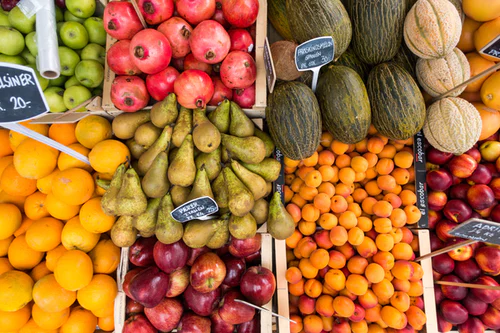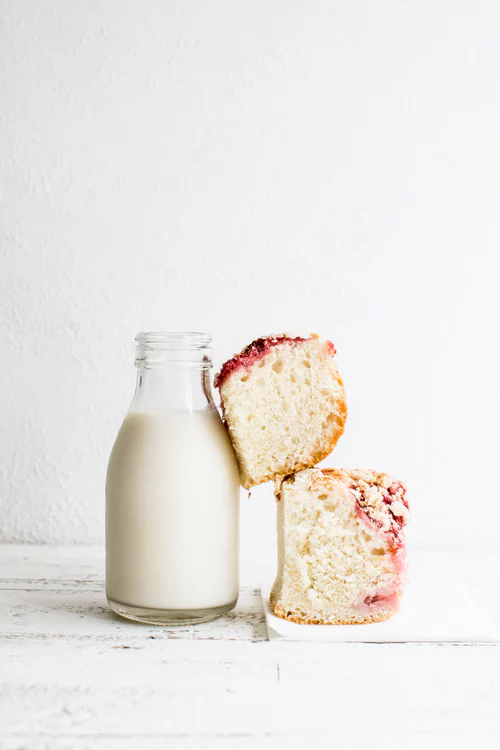# Using Algebra to Find Real Life Solutions

## Translating problems into algebra

Algebra is extremely useful to help solve problems that involve generalising or finding an unknown variable.

You can use algebra in many ways in the real world, often this means you will need to create an equation and then solve it.

For example we can use algebra to help use solve problems like this.I am going to the shops and I want to buy 5 apples and 3 pears. I ended up spending £5 and each pear cost me 30 pence, how much did the apples cost me?

So, the total cost was £5 , that is equal to the price of 5 apples,(I’ll call this price 5a) and 3 pears, which we know cost £0.30.

Therefore £5 = 5a +3(0.30) with this equation I can work out the price of the apples!

£5 - £0.90 = 5a

£4.10 = 5a

£0.82 = a

So the price of one apple is equal to £0.82.

## Solving real life problems with simultaneous equations

For more complex problems, or for problems where we don’t know a variable we might need to use a simultaneous equation. For example:I went to the shops on Tuesday and I bought 2 loaves of bread and 3 bottles of milk. It cost me £3.50. On Wednesday I went to the shops and bought 1 loaves of bread and 2 bottle of milk and it cost me £2.15. How much is one bottle of milk and how much is one loaf of bread?

To solve this I need to create a simultaneous equation.

Tuesday 3m +2b=£3.50

Wednesday 2m + b=£2.15

As before in Solving Simultaneous Equations Algebraically we need to make one variable in each equation equal. We can do that by multiplying Wednesday’s equation by 2:

Tuesday 3m +2b=£3.50 x1 = 3m+2b=£3.50

Wednesday 2m + b=£2.15 x2 = 4m +2b=£4.30

To find one variable because the signs are the same we need to take the equations away from each other.

It doesn’t matter which order I take them away:

You can take Tuesday- Wednesday or Wednesday- Tuesday

4m +2b=£4.30 3m+2b=£3.50

3m+2b=£3.50 4m +2b=£4.30

m +0= 0.80 -m +0 =-0.80

Therefore m=£0.80 Now we can work out b

3 (0.80)+2b=£3.50

£2.40 +2b = £3.50

2b = £1.10

b= £0.55

So one loaf of bread is £0.55 and one bottle of milk is £0.80. We can check this by substituting out values into the original equations.